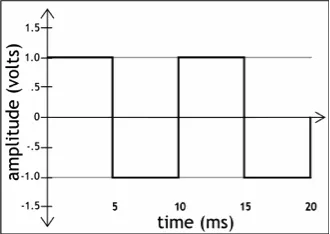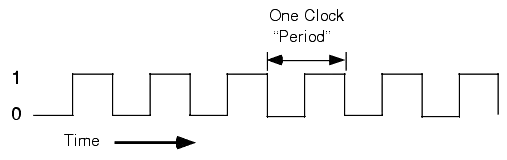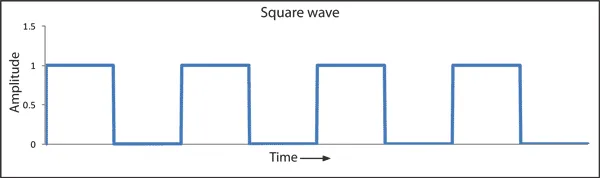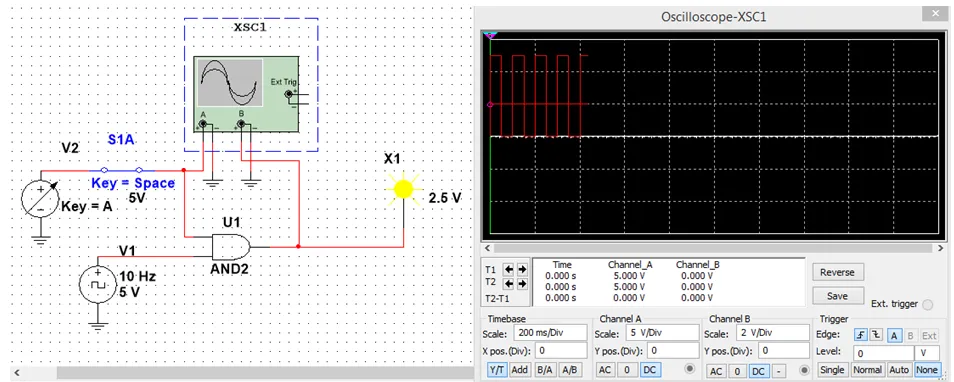# Logic Design - Waveforms and ClocksHello it's me! This time in Logic Design we will talk about Waveforms and Clocks that will help us understand some stuff in Circuits much better. Using Clocks we will be able to automate Circuits, so that we can test our Circuit out for each and every Input Combination, to see if the Output is correct. To do this tho we will need to have some knowledge in Waveforms, and all that stuff will lead us in Latches and Flip-Flops that we will talk about in the next Logic Design Post. So, let's get started then!

# Waveforms:

Waveforms are shapes and forms of signals. There are many different Types of them. The most common ones are the periodic signals, where time, wavelength, amplitude and phase are the main attributes that define them.

We split them into:

• Sine Waves (that follow the amplitude of the trigonometric sine function)
• Square Waves (that periodically switch from a to b and vise versa, so that we have square shapes)
• Triangle Waves (that periodically switch between a and b and vise versa going in a straight line, so that it forms a triangle shape)
• Sawtooth Waves (that periodically switch between a and b going only from a to b in a straight line directly and getting directly into a again or the opposite, forming a saw shape)

We can also have non periodic waveforms and the most common one I will use for testing looks almost like an square wave, but we will take the control of change using switches and not a Clock, but an constant energy source!

The device that prints out waveforms for a specific line in our Circuit is called Oscilloscope. We will get into when we talk about MultiSim!

# Clocks:

Clocks are Generators of Waveforms! We use them to oscillate between the high(1) and low(0) voltage state in periodic timing. In our Circuits we will mostly use those that form Square Waves and maybe Sine Waves! We can define the Amplitude and the Frequency of such an Clock. The Amplitude is the high voltage value that we mostly put at 1 and the Frequency defines how often it should change value! We can also setup in which Phase of the Period the Clock should start out! (You will understand all that much better when we get into MultiSim Implementation and some stuff like Phasing will get more clear when we get into VHDL)

So, to recap all that. A Clock is a device that generates signal waveforms. We mostly use Square Waves and we have 3 Attributes called Amplitude (mostly 1), Frequency (how often it switches between 0 and 1) and Phase (fraction of the period the clock should start) that define such an Clock!Clocks are a part of any Sequential Circuit and we will use them for Input Combination testing in every Circuit to make things easier! In Sequential Circuits a Clock Signal is used in 2 different ways. We either have a synchronization in every rising edge or in every falling edge (we will get into more next time).

Let's now get into how we use Clocks to test each and every Input Combination.

# Testing All Input Combinations:

When testing out each an every Input Combination of an Logical Circuit the Square Wave in the best option!We will have Clocks and Constant Sources in our Circuit and check the Output value for testing each Input Combination, or we can also see what kind of value some Line in between the Circuit has.

Example:

Suppose we want to test an AND Gate. We can use 1 Clock and a Constant Source that has a Switch. We could also use 2 Clocks, where one has half or double the frequency of the other so that we end up with all combinations. But, I will stick with the first one.

The Circuit and the Output of the Oscilloscope in MultiSim looks like this:You can see that when having the Switch for the first Input Active the Output is 1 only when the Clock Signal is 1 too! That makes sense, cause we are talking about an AND Gate that gives us 1 only when both Inputs are 1. So, our Outputs follows an Square Waveform, that has the same frequency as our Clock.

Hope you enjoyed this post!

This was some Theory that I had to split from the next Logic Design post, cause else the next one would become too big (and I don't want that). Next Time in Logic Design we will get into Latches and Flip-Flops, so that we finish the Building Blocks of Sequential Circuits, to get into them after that!

Bye!

H2
H3
H4
3 columns
2 columns
1 column
Join the conversation now